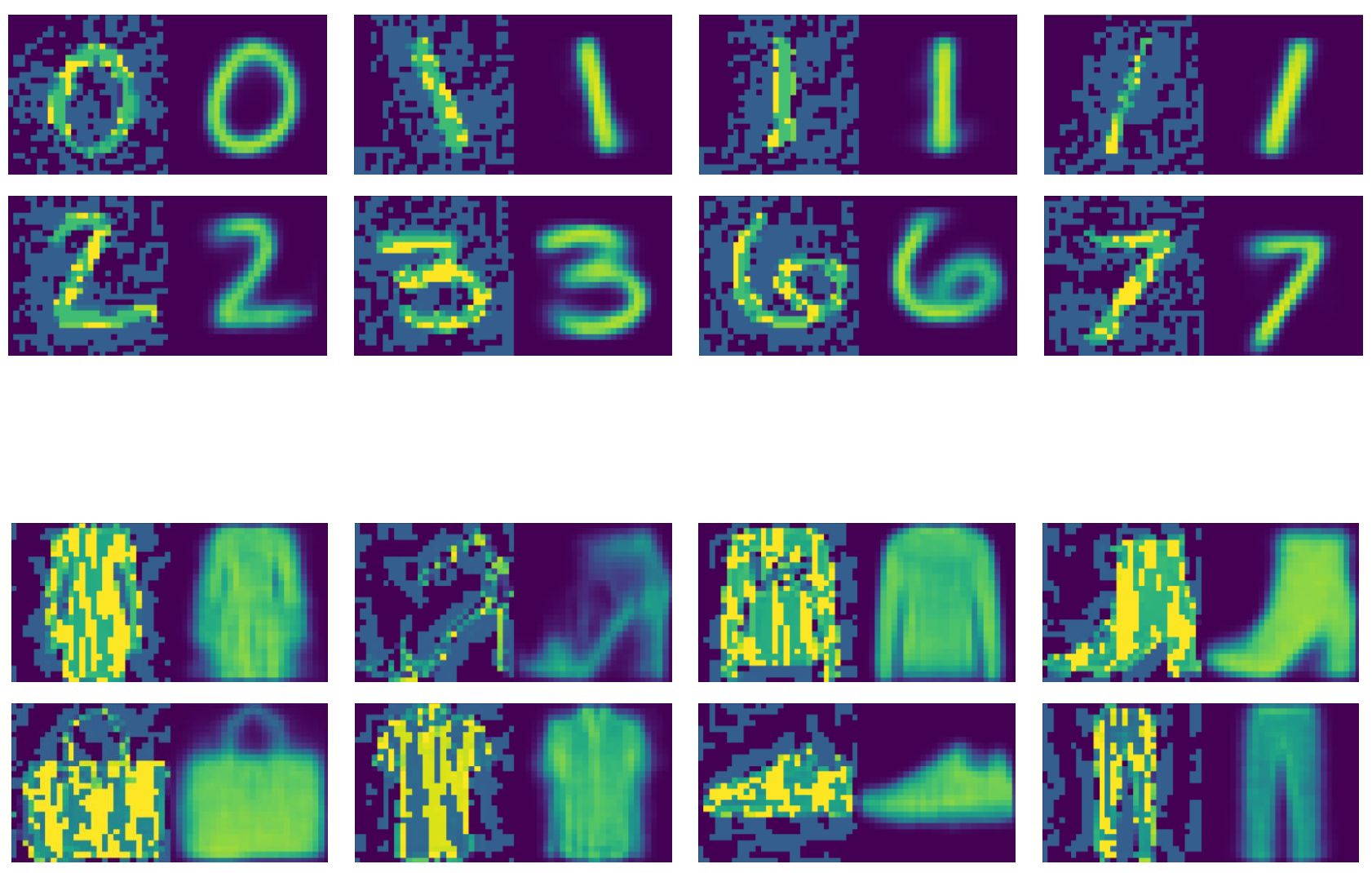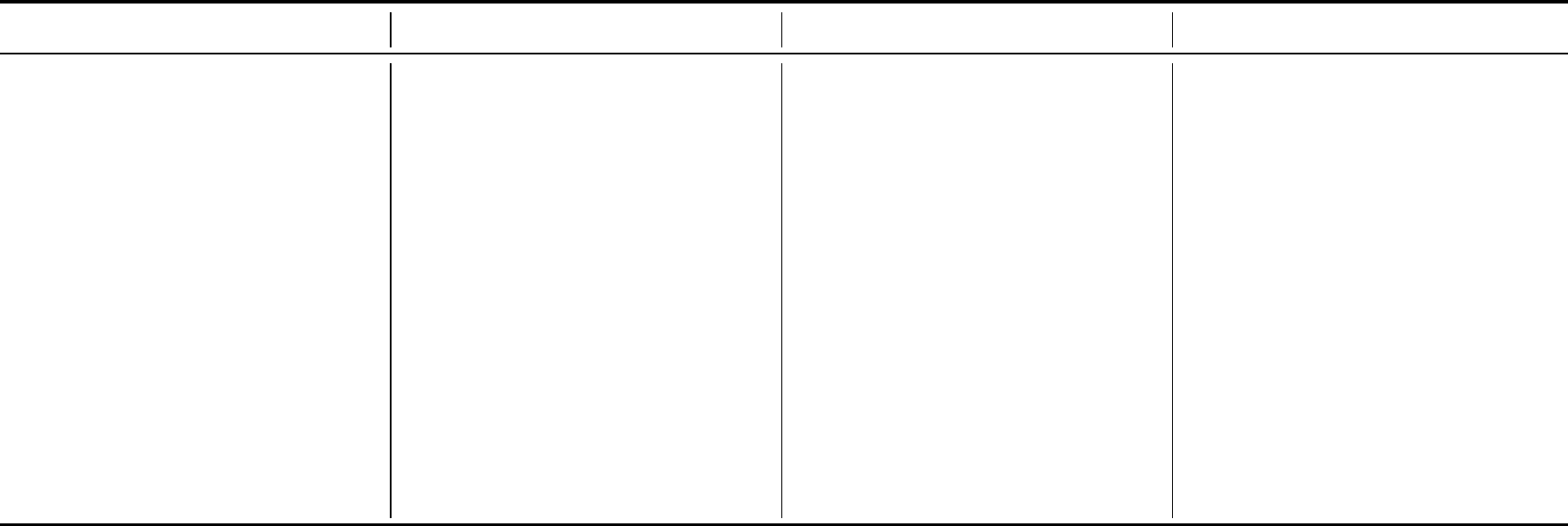Supplementary Materials
PuVAE: A Variational Autoencoder to Purify Adversarial Examples
Anonymous Authors
A Puriﬁed Images from PuVAE
Figures 1 and 2 are the visualizations of the experimental results on the MNIST and Fashion-MNIST datasets respectively. In
each image, the left is the image attacked with FGSM(0.3), and the right is the puriﬁed result of the left image using the PuVAE.
In both datasets, the adversarial noise is selectively removed while preserving the original shape of the image. Particularly, the
PuVAE puriﬁes adversarial examples while maintaining the angle of the number 1 in the ﬁrst row of Figure 1.
Figure 1: Examples of puriﬁed images from PuVAE on MNIST dataset.
Figure 2: Examples of puriﬁed images from PuVAE using Fashion-MNIST dataset.B Architectures of Target Classiﬁers
The target classiﬁers are convolutional neural networks, and the details are shown in Table 1. We used A and B architectures for
MNIST and Fashion-MNIST, and C and D architectures for CIFAR-10.
Table 1: Neural network architectures used for target classiﬁers
A B C D
Conv(64, 5×5, 1) Conv(128, 3×3, 1) Conv(64, 5×5, 1) Conv(128, 3×3, 1)
ReLU ReLU ReLU ReLU
Conv(64, 5×5, 2) Conv(64, 3×3, 2) Conv(128, 5×5, 1) Conv(256, 3×3, 1)
ReLU ReLU ReLU ReLU
Dropout(0.25) Dropout(0.25) Conv(256, 5×5, 2) Conv(512, 3×3, 2)
FC(128) FC(128) ReLU ReLU
ReLU ReLU Dropout(0.25) Dropout(0.25)
Dropout(0.5) Dropout(0.5) FC(512) FC(512)
FC(10) + Softmax FC(10) + Softmax ReLU ReLU
Dropout(0.5) Dropout(0.5)
FC(256) FC(10) + Softmax
ReLU
FC(10) + Softmax